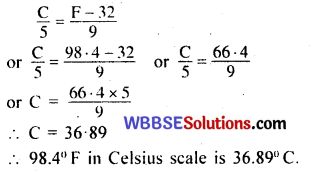# WBBSE Class 7 Science Solutions Chapter 1.1 Heat

Detailed explanations in West Bengal Board Class 7 Science Book Solutions Chapter 1.1 Heat offer valuable context and analysis.

## WBBSE Class 7 Science Chapter 1.1 Question Answer – Heat

(Page no. 2)

Both of the thermometers are of same type. Bulbs of both the thermometers are – immersed into the same liquid as shown in the figures 1 and 2.

a Do you think that the height of the mercury columns in the two thermometers in figure 1 have been shown correctly? Justify your answer.
Yes, because the height of the mercury columns in the both the thermometers are same.Do you think that the heights of the mercury columns in the two thermometers in figure 2 have been shown correctly ? Give reason for your answer.
No, thermometers are different and ‘B’ thermometers are different. This is impossible because they immersed in same liquid.

Write the numbers chosen by you in the exact portions given below. (Page – 4)(Page no. 5)
Fill in the following table by taking date from Table -1 and Table -2.(Page no. 6)

Now say how many divisions are there from θ° to C and 32° to F.
50 and 90 divisions respectively.Now calculate 40°C in terms of degree Fahrenheit.(Page no. 7)

Now in a bucket water of 15°C is taken and in a tub water of 9°C is taken. Now say, what will happen after mixing of water of bucket and that of tub?
The mixed water is less warm than the water in tub [ ]
Mixed water is more warm than water in bucket. [✓]

(Page no. 8)

Fill in the following blanks
The rise in temperature of the water in the first container = 50°C.
The rise in temperature of the water in the second container = 75°C.

Water in which container has observed more heat to obtain the temperatures stated above?
The second container.
Now the two containers with water at 50°C and 75°C are kept at room temperature (25°C).
After sometime water of the two containers will separately reach room temperature. The irst container with decrease by (50-25)°C = 25°C and the second container will decrease by (75-25)°C = 50°C.water of which container will lose more quantity of heat?
The second container.

(Page no. 9)

In which case will water absorb more heat in going from a temperature of 25°C to 50°C ? Will it be the case of one glass of water or twenty glasses of water ?
Twenty glass of water.

Fill in the table given below :- (Page no. 10)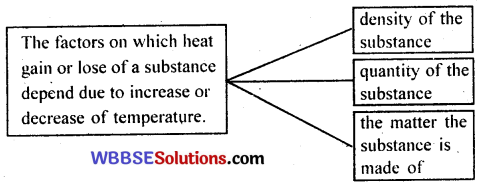Now fill up the following table : (Page no. 12)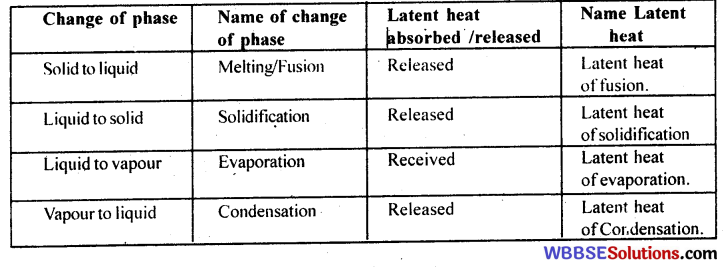Now see whether you can explain the incidents given below. (Page no.13)

Why there is a cool sensation after bathing ?
When we bath. some water evaporates taking the latent heat from our body. So our body loses some amount of heat. The temperature of our body becomes less than adjacent surrounding. So we feel a cool sensation after bathing.

Why the floor becomes cool when it is mopped with water?
When floor of a room is mopped with water, the water evaporates taking the latent heat from the floor and also from the adjacent surroundings. So the floor loses heat and becomes cool.During summer if all the doors and windows are kept open and wet curtains are hung, the room remains moderately cool. Explain.
During summer if all the doors and windows are kept open and wet curtains are hung. the water from the curtain evaporates slowly. In this process the required latent heat is taken by water from room. so the room become cool.

Influence of heat and temperature on living being.
Influence of heat and temperature is observed on the variation of stucture, nature and lifestyle of living being. Animals of winter zone are more hairy than the animals of summer zone. In a hot day more sweating from the human body and panting of dog is observed. All of them keep the body cool. Again to keep the body warm there is dense fur on the body of Polar Bear. The penguins hug each other for the same reason. Plats are dried up in very hot condition. Again cameleon. cold blooded animals like snake enjoy the heat of the sun or heat of the sand. These phenomena occur as a result of the influence of heat.

Let’s see, what people do when the temperaturerises. (Page no. 14)
Word troves : breathing. food intake, perspiration, work.

1. They raise perspiration rate. 2. Breathing rate is increased. 3. The diameter of Blood vessel is increased. 4. Amount of food intake is decreased. 5. Aversion to work and lethergy are seen.

What happens in the body of human beings when temperature of the body is decreased? Write that with the help of following word trove.
Word troves : trembling, food intake, sweating, hairs.
1. Trembling occurs. 2. The rate of food intake decreases. 3. Excessive sweating increases. 4. The hairs stand on end.

Discuss yourselves about phenomena where influence of heat is observed on living organisms of atmosphere and write in the space given below.
Generally white flowers blessom in the night in less temperature, the flowers with coloured petals bloom in daytime, sunflower blossoms when sun’s ray fall on it, during winter season merrigold blooms only in cold temperature.

### Class 7 Science Chapter 1.1 Question Answer West Bengal Board –Heat

Multiple Choice Questions & Answers : (1 mark for each question)

Question 1.
Celsius scale is divided into-
i) 80 parts
ii) 180 parts
iii) 100 parts
iv) 120 parts
iii) 100 partsQuestion 2.
Freezing point of Fahrenheit scale is –
i) 32° F
ii) 0°F
iii) 180° F
iv) 80°F
i) 32° F

Question 3.
To make thermometer quickly effective-
i) Shape of the bulb should be large
ii) Shape of the bulb should be small
iii) Bulb should be made round in shape
ii) Shape of the bulb should be small

Question 4.
1 Calorie =
i) 4.18400 joules
ii) 1.18400 joules
iii) 2.18400 joules
iv) 3.18400 joules
i) 4.18400 joules

Question 5.
The amount of heat required to change the temperature of unit mass of the substance by one unit is called-
i) latent heat
ii) heat
iii) specific heat capacity
iii) specific heat capacity

Question 6.
Temperature of substance A is 100°F and temperature of substance B is 100° C. Then say which of the following is correct.
i) B substance has more heat
ii) A substance has more heat
iii) Two substance has same heat
i) B substance has more heatQuestion 7.
Which of the following substances have highest specific heat capacity-
i) Alcohot
ii) Kerosene
iii) Water
iii) Water

Question 8.
The instrument by which heat absorbd or heat released is measured is called-
i) Barometer
ii) Thermometer
iii) Caloriemeter
iii) Caloriemeter

Question 9.
Heat is a from of-
i) substance
ii) energy
iii) absorber
ii) energy

Question 10.
The amount of heat energy required to raise the temperature of 1 ~g of water by 1°C is called-
i) 1 J
ii) 1 K cal
iii) 1 Calorie
iii) I Calorie

Question 11.
Water stored in earthen pots gets cooled due to-
i) evaporation
ii) boiling
iii) freezing
i) evaporation

Question 12.
If in two same type of kettle equal quantity of water and milk are boiled by same time keeping side by side the-
i) Water and milk would began to boil at the same time
ii) Milk will boil quicker than water
iii) Water will boil faster than milk
ii) Milk will boil quicker than waterQuestion 13.
Change of vapour to liquid is called-
i) melting
ii) evaporation
iii) boiling
iv) condensation
iv) condensation

Question 14.
In SI system specific heat capacity of water is-
i) 4.2 Joule
ii) 4200 J/Kg
iii) 42 J/Kg
ii) 4200 J/Kg

Question 15.
Change of solid to liquid is called-
i) melting
ii) evaporation
iii) melting
iv) cordensation
i) nelting

Fill in the blanks : (1 mark for each question)

1. All the substances do _____ a definite melting point.
not have

2. When pressure is increased, melting point of ______ decrease.
ice.3. In a definite pressure pure crystal shaped substances have _______ melting and freering point.
same.

4. When moulded iron is solidified its shape ______.
increase.

5. Wax has _______ definite melting point.
no.

6. Ether is a _____ liquid.
volatile

7. If heat is the reason then temperature is its _____.
result.

8. 0°C = – °F
32°.

9. Clinical thermometer should not be immersed in ______ water.
Boiling.10. Heat is a form of ______.
energy.

11. The degree of hotness or coldness of a body is called _______.
temperature.

12. When two bodies of different temperatures are kept in contact, the hot body loses heat and the cold body gains lieat, till their temperatures are ______.
same

13. The boiling process takes place ove ______ liquid.
entire.

14. In CGS system the unit of heat is ______ .
calories

15. Heat always flows from ______ temperature to ______ temperature.
higher, lower.16. Heat is a _______ quantity.
scaler.

17. Normal temperature of a human body is 98.4°F. In celcius scale it is _______.
36.89°C.

18. Hahrenheit is divided into _______ equal parts.
180°

19. _____ is responsible for the change of state of a substance.
latent heat.

20. ____ has a very high latent heat of vaporisation.
water.

State whether True or False : (1 mark for each question)

1. The floor becomes cold after mopping it with water.
2. Spirit evaporates very slowly.
3. Change from liquid to solid is calied solidification.
4. The unit used in SI system to measure heat is calorie.
5. The change of phase of a substance from solid to liquid is called melting.
6. If water is kept in an earthen pitcher, it becomes cool.
7. The leaves of babul and radhachura ere open at night.
8. When temperature of human body decreases trembling occures.
9. When body temperature rises in people breathin, rate is increased.
10. Plants are dried up in very cold condition.
1. true
2. false
3. true
4. false
5. true
6. true
7. false
8. true
9. true
10. falseMatch the following: (1 mark for each question)

a) Match the column A with column B.

 Column A Column B a) Liquid to solid– i) after bathing b) Vapour to liquid– ii) snake c) Cold-blooded animal– iii) volatile substance d) Spirit is a iv) open in daylight e) Cool sensation is felt v) solidification f) 0° C to 100°C– vi) are hairy g) Latent heat is required for vii) condensation h) Animals of of cold region viii) Celsius scale i) The leaves of some trees ix) evaporation

a) I.iquid to solid — solidification.
b) Vapour to liquid — condensation.
c) Cold-blooded animal — snake
d) Spirit is a volatile substance.
e) Cool sensation is felt after bathing.
f) 0° C to 100° C – Celsius scale.
g) Latent heat is required for evaporation.
h) Animals of cold region are hairy.
i) The leaves of some trees open in daylight.

Very Short Questions & Answers : (1 mark for each question)

Question 1.
What is the SI unit of temperature?
The SI unit of temperature is Kelvin (K).Question 2.
Which liquid has the highest specific heat capacity?
Water has the highest specific heat capacity.

Question 3.
What are the limitations of Celsius scale?
In Celsius scale lower limit is 0°C and upper limit is 100°C.

Question 4.
How many divisions are there between upper and lower limit of Fahrenheit scale?
In Fahrenheit scale there are divisions Upper limit – lower limit = 212°F-32°F=

Question 5.
Is heat vector quantity?
No, heat is scaler quantity.

Question 6.
0°C or 0° F which one is lesser?
between 0°C and 0°F, 0°F is lesser.

Question 7.
In CGS system what is the unit of temperature?
In CGS system the unit of temperature is degree celsius.

Question 8.
What is the heat r.easering property of mercury thermometer?
The measuring property of thermometer is its expansion due to heat.Question 9.
1 Calorie = how many Joule?
1 Calorie =4.2 Joule.

Question 10.
What do you mean by primary difference of a thermometer?
The difference between the upper limitation and lower limitation of a thermoneter is called the primary difference of a thermometer.

Question 11.
What is the amount of heat given out by a body?
Heat given out by a body = mass of the body × specific heat capacity of the body × fall in temperature of the body.

Question 12.
What is the amount of heat absorbed by a body ?
Heat absorbed by a body = mass of the body × specific heat capacity of the body × rise in Temperature of the body.

Question 13.
How could you find out specific heat capacity of a substance?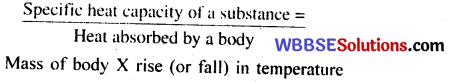Question 14.
What is the unit of specific heat capacity in CGS system?
In CGS system the unit of specific heat capacity is Calorie / gran / °C.

Question 15.
What is melting?
Change of a substance from solid to liquid state is called melting.Question 16.
What is solidification?
Change of a substance from liquid to solid state is called solidification.

Question 17.
What is evaporation?
Change of a substance from liquid to vapour state is called evaporation.

Question 18.
What is condensation?
Change of a substance from vapour to liquid state is called condensation.

Question 19.
What is latent heat of a substance?
Latent heat of a substance is defined as the amount of heat required to change the state of the substance without any change in temperature.

Question 20.
What is latent heat of fusion?
The latent heat of fusion is the amount of heat required to change a substance from the solid state to its liquid state at its melting point without any change in temperature.

Question 21.
What is the latent heat of the fusion of ice?
The latent heat of the fusion of ice is 80 calorie / gram.Question 22.
Name a volatile substance.
Sprit or ether is a volatile substance.

Question 23.
What is vaporization?
Vaporization is a transitional phase of a compound or an element and it occurs during the boilling or sublimation process.

Question 24.
What is evaporation?
Evoporation is nothing but a type of vaporization which mostly occurs at temperatures below the boiling point.

Question 25.
What is necessary for vaporization?
Latent heat is necessary for vaporization.

Question 26.
What a dog do to keep its body cool?
A dog projects its tongue out and pants to keep its body cool.

Question 27.
What is the unit of measuring heat in SI system?
In SI system heat is measured in joule.

Question 28.
Name another unit other than joule which is used to measure heat.
Other than joule heat is measured in calorie.Question 29.
What is used to measure fever?
Clinical thermometer is used to measure fever.

Question 30.
What is used inside thermometer?
Mercury is used inside the thermometer.

Question 31.
In what degree celsius water converts into ice?
In 0° celsius water converts into ice.

Short Questions & Answers : (2 marks for each question)

Question 1.
What is heat? Write down its units.
Heat is a form of energy which causes a sensation of hotness and coldness and which can be transferred from a hotter body to a cooler body.
Units of heat are – In CGS system it is calorie and in SI system it is joule. I Calorie = 4.2 joule.

Question 2.
What is temperature? Write down its units.
Temperature is the degree of hotness or coldness of a body. Temperture is a relative measure, or indication of hotness or coldness.
Units of temperature are – In CGS system it is degree celsius (0°C) and in SI system it is Kelvin (K).

Question 3.
What is thermometer? What is mercury thermometer?
Thermometer is an instrument which measures the temperature of a substance perfectly. A thermometer in which mercury is used for measuring temperature is called mercury thermometer.Question 4.
What is Celsius scale?
The thermometer scale which was invented by the Swidish as tranomer Anders Celsius is named after him. He devised the inverted form of this scale – ice point 0°n and steam point 100°. The scale is divided into 100 parts. Every part is called 1°C.

Question 5.
What is Fahrenheit scale?
Fahrenheit scale is a temperature scale in which the temperature of boiling water is taken as 212 degrees and the temperature of melting ice is taken as 32 degrees. It was invented by Gabriel Fahrenheit a German physicist. The scale is diveded into 180 parts and each part is taken as 1°F.

Question 6.
What do you mean by 1 calorie of heat? Is it a vector quantity?
The amount of heat energy required to raise the temperature of 1 gram of pure water by 1°C is called 1 caloric. Heat is has only magnitude but no direction so it is a scalar quantity. It is not vector quantity.

Question 7.
What is specific latent heat ?
Specific latent heat of a substance is defined as the amount of heat required to change the state of unit mass of the substance without any change in temperature.Question 8.
Name three factors that determine the quantity of heat absorbed by a body.
The quantity of heat gained by a body depends on the following three factors.
a) Mass of the substance
b) Nature of the substance
c) Rise in temperature of the substance.

Question 9.
What is the principal of calorimetry ?
The principal of calorimetry states that if two bodies at different temparatures are placed in contact with each other, the hot body will lose heat till they reach a common temperature.

Question 10.
At what temperature the reading of Fahrenheit will be twice than Celsius scale?
Taking C = x and F = 2x.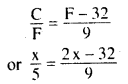or, 9 x = 10x – 160 or, 9x – 10 x =-160
or, – x = – 160 or x = 160
∴ Required temperatures are 160°C or 320°F

Long Questions & Answers : (3 marks for each question)

Question 1.
What are the fixed points of a thermometer? What do you mean by lower fixed point and upper fixed point of a thermometer?
Two definite temperatures are taken as upper and lower limits to make a scale of a thermometer. There two definite limits are called fixed points of a thermometer.

Lower fixed point – In a definite temperature and in a definite atınospheric pressure when ice changes into water. then this temperature is called lower fixed point.

Upper fixed point – In a definite temperature and in a definite atmospherie pressure when water begings to boil and change into vapour, then this temperature is called upper fixed point.Question 2.
What is relation between Celsius scale and Fahrenheit scale ?
100 division in Celsius scale is equal to 180 division in Fahrenheit scale.
Then 1 division in Celsius scale = $$\frac{180}{100}$$ division in Fahrenheit scale.
C division in Celsius scale =$$\frac{180}{100}$$ divisions in Fahrenheit scale.
Then we can write $$\frac{180}{100}$$ = F-32 or $$\frac{9}{5}$$=F-32 or c=(F-32)

Again $$\frac{9C}{5}$$ = F-32
then $$\frac{C}{5}$$ = $$\frac{F-32}{9}$$
C =$$\frac{5}{9}$$(F-32)

Question 3.
State the difference between Heat and Temperature.

 Heat Temperature 1. Heat is a form of energy. 1. Temperature is the degree of hotness or coldness of a body. 2. Heat is the reason of temperarure. 2. Temperature is the result of heat. 3. Units of heat in CGS system is calorie and in SI system is Joule 3. Units of temperature in CGS system is degree celsius and in SI system is Kelvin. 4. Heat is measured by an instrument called calorimeter. 4. Temperature is measured by thermometer.

Question 4.
Explain why water in an earthen pitcher remains cool.
Water in earthen pitcher remains cool. Water in earthen pitcher remains cool. In fact, numerous capillary holes are there, in the wall of earthen pitcher. Through the holes a little amount of water comes out from the pitcher. Then envaporation takes place. The process needs latent heat. Then the water on the wall absorbs requirite latent heat the pitcher and water in it. As a result the pitcher and water in side it loses that heat and become cool.

Question 5.
Why cooling sensation is felt when ether or sprit is poured on hand?
In fact, spirit or ether is a volatile compound (this type of substance evaporates very quickly). Latent heat is required for evaporation. The spirit or ether absorbs latent heat fron the surrounding also and from the hand. Therefore, the particular position of hand loses heat, that is why, when spirit or ether is poured on hand, cooling sensation is felt at that spot.Question 6.
State the difference between evaporation and boiling.

 Evaporation Boiling 1. Evaporation taks place at all temperatures. 1. Boiling takes place at the boiling point of the liquid. 2. Evaporation is a slow and slient process. 2. Boiling is a fast and violent process. 3. Evaporation takes place only at the surface of the liquid. 3. Boiling takes place over the entire mass of the liquid. 4. Evaporation causes cooling. 4. Boiling does not cause cooling.

Question 7.
Normal temperature of a body in clinical thermometer is 98.4°F, how much it will be in Celsius scale ?